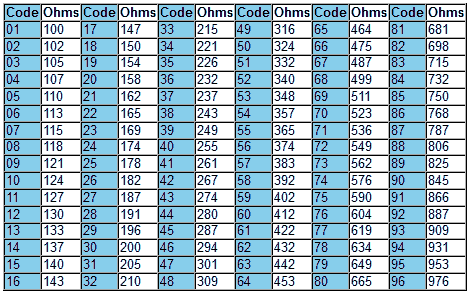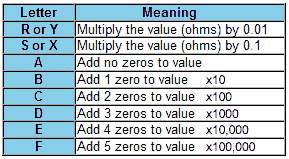# Surface Mount Resistors

• After studying this section, you should be able to:
• • Identify the values of surface mount (SMT) resistors.
• 3 and 4 digit codes.
• EIA E-96 code.

## Identifying the Values of Surface Mount Resistors

Surface Mount Technology (SMT) Resistors are available in a range of standard packages (shape and size) agreed by the Electronics Industry Alliance (EIA) through the Solid State Technology Association, formerly known as Joint Electron Device Engineering Council (JEDEC).

These packages are given identifying numbers derived from the (approximate) ‘footprint’ size of the component measured in inches, i.e. the area the component occupies on a printed circuit board. The packages listed below are in common use for resistors and capacitors.### Table 2.3.1 SMT packages

Because surface mount resistors are so small, there is not enough space for colour code bands. The markings used to give the value of the resistor consist of 3 or 4 letters or numbers that may be easier to read with a magnifying glass.

Reading the codes is made more complicated because there are number of different codes in use. Most common is a 3 number code that works in a similar way to the colour code bands on wire-ended resistors.

The first two numbers give the first two digits of the resistor's value whilst the third digit gives the number of zeros (or multiplying factor).### Fig. 2.3.1 SMT resistors with Three Digit Code

For example:

A resistor marked 332 is 3300 or 3.3KΩ (or 3K3 with the K replacing the decimal point). A resistor marked 475 is 4,700,000 or 4.7MΩ (or 4M7 with the M replacing the decimal point).

For resistors less than 100 Ohms, the last figure will be 0 indicating NO zeros. Therefore 33 ohms would be marked 330 ( i.e. thirty three and no zeros) although some resistors may be marked 33R (to avoid confusion!).

A 330 ohm resistor would be marked as 331 (thirty three followed by one zero).

What if the value is even lower, 4.7ohms for example?

Then the decimal point is replaced by letter R to give 4R7.

There is also a 4-digit code in use for resistors with low tolerances of +/-1% or less that gives the 3 digits of the value and uses the fourth digit for the number of zeros (the multiplier).

Using this code a 10 ohm resistor would be marked 10R0, 100 ohms is marked 1000, and a 1K ohm is 1001 etc.

### EIA-96 Coding Scheme

An alternative scheme to the 3 and 4 digit codes is the EIA-96 code, which uses two numbers and a letter to refer to any of the 96 standard values in the E96 range.

Each 2 digit number code refers to one of the 96 values in the E96 +/-1% tolerance range of resistors shown in Table 2.3.2. These digits are followed by a letter to indicate one of the eight multipliers shown in the EIA_96 Letter Multiplier table 2.3.3.### Table 2.3.2 SMT E96 Numerical Codes### Table 2.3.3 EIA-96 Letter Multiplier Codes### Fig. 2.3.2 EIA-96 Coded1M58 +/-1% Resistor

For example the resistor shown in Fig. 2.3.2 marked 20E would be 158Ω (20 from Table 2.3.2) multiplied by 10,000 (E from Table 2.3.3) = 1.58MΩ (1M58 +/-1%).